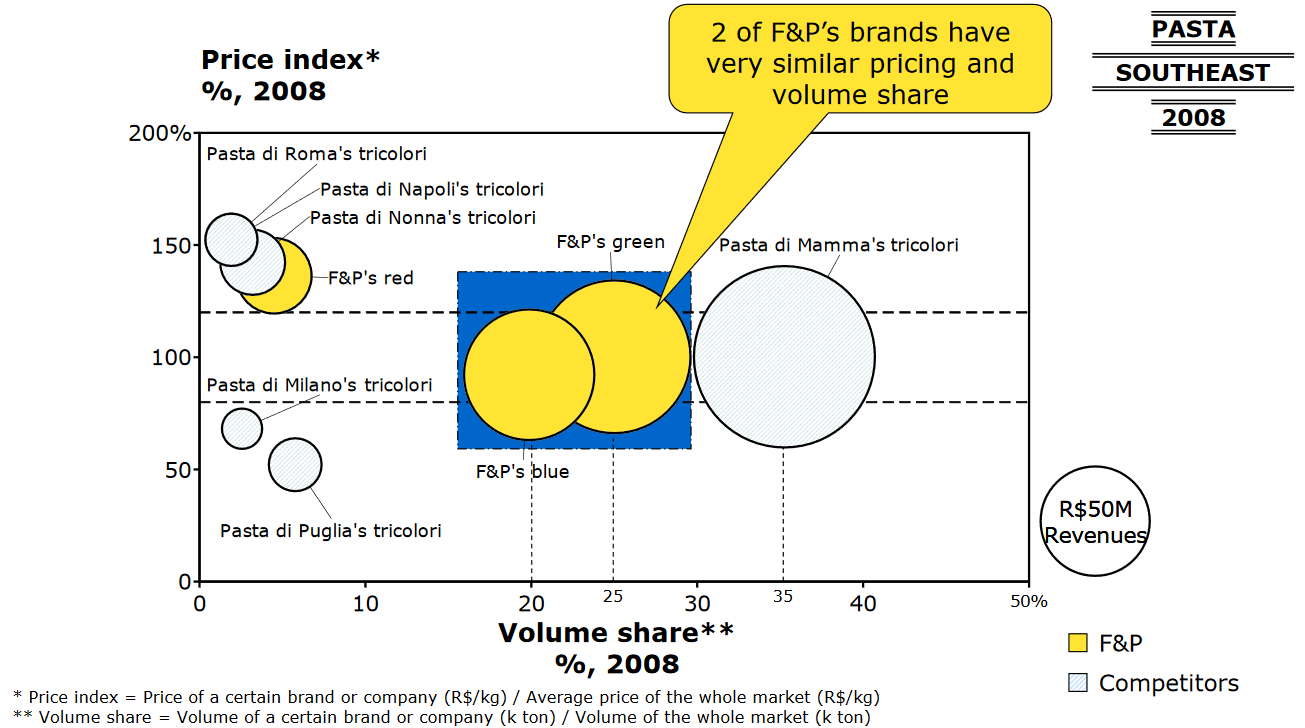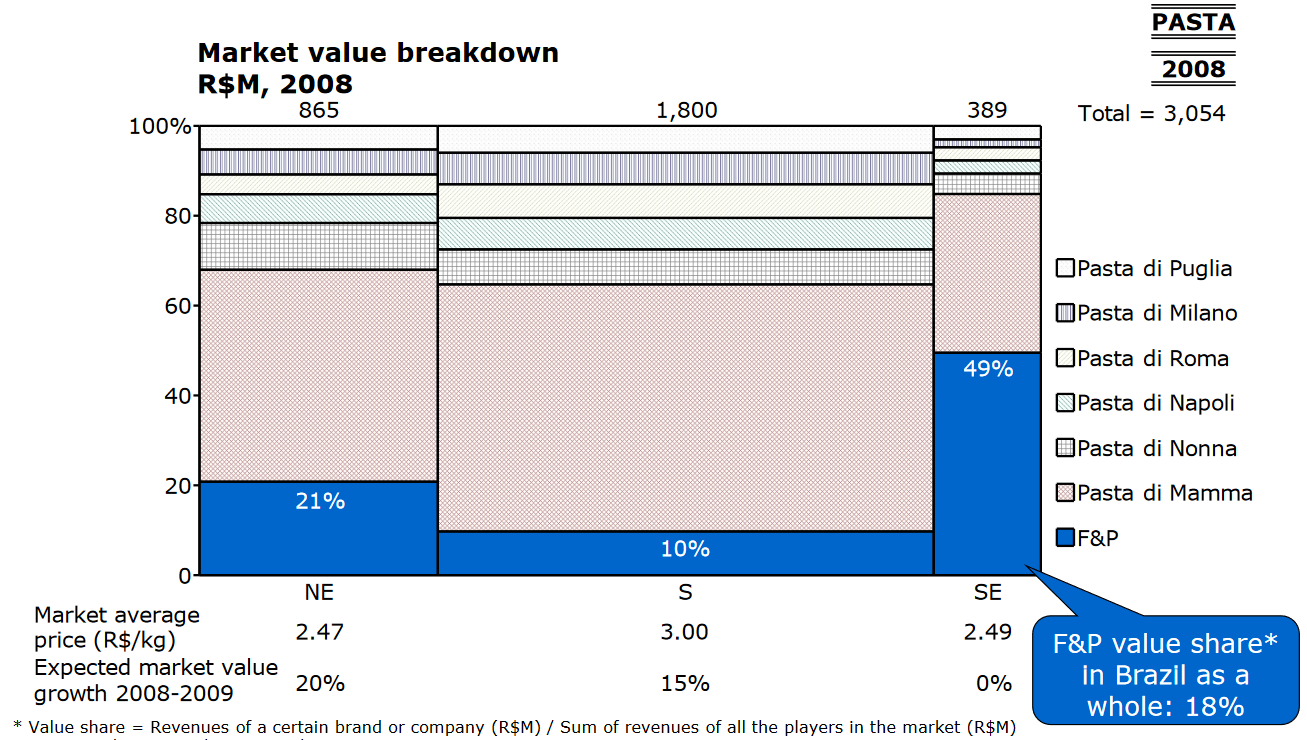# How to calculate volume after merge from a Price Index - Volume Share chart

Recent activity on Mar 25, 2019
1.8 k Views

Hi! I'm doing a sample case and I'm not quite sure how to find the volume after the merge of F&P's green and blue.

I know the correct answer is 70 but how do I get there?

My guess is that it will be the sum of both areas but I'm don't know what is the best way of getting this.

Any help would be great!• Date ascending
• Date descending

1. We can calculate Revenue per kg for F\$P blue and green ( they are 100% price index with avg. market price 2.49, wich means 2.49 per kg. ). (won't consider red for simplicity )
2. We can calulate their total volume, dividing total their total revenue (which we can get from their volume share int SE = 0.49*389 = 191 ) by revenue per kg (2.49) - we get 76.4M
3. Approx 10% of volume attributes to red (from first graph), which means volume after merge is ~70M

Hi Alexander

The only other slide about Pasta is this one for value breakdown

I thought about multiplying 49% * \$389M to get F&P's total value in Southeast (the merger in question is only in the SE region) and try to use that to know how much would be 20% of the whole market for F&P's blue and 25% for F&P's green.

However 49% * \$389M is ~\$190M, way over the \$50M total revenue from the original chart. And I still don't have any information about how many tons this would be

How can I use this info to get a value in tons? I don't see anything I can do with these slides